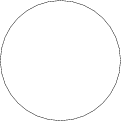# User:Ulf Rehmann/Test3

(diff) ← Older revision | Latest revision (diff) | Newer revision → (diff)

$$e^{2\pi i} - 1 = 0$$

$$A,B,C, D, E, F, G$$

XXXYYY Additional text

Additional text $$(a+b)$$ $$(x-y)$$

no [[wiki]] ''markup'' $$(x-y)$$ $$(x-y)$$ $$(x-y)$$ $$(x-y)$$

How to Cite This Entry:
Ulf Rehmann/Test3. Encyclopedia of Mathematics. URL: http://encyclopediaofmath.org/index.php?title=Ulf_Rehmann/Test3&oldid=49281